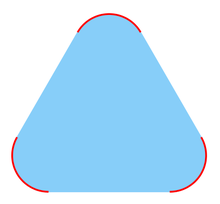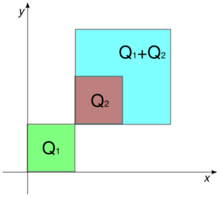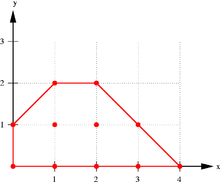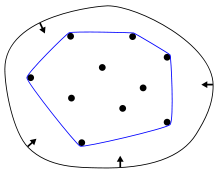# Convex hull

Convex hullThe convex hull of the red set is the blue convex set.

In mathematics, the convex hull or convex envelope for a set of points X in a real vector space V is the minimal convex set containing X.

The convex hull also has a linear-algebraic characterization: the convex hull of X is the set of all convex combinations of points in X.

In computational geometry, a basic problem is finding the convex hull for a given finite set of points in the plane.

## Existence of the convex hull

To show that the convex hull of a set X in a real vector space V exists, notice that X is contained in at least one convex set (the whole space V, for example), and any intersection of convex sets containing X is also a convex set containing X. It is then clear that the convex hull is the intersection of all convex sets containing X. This can be used as an alternative definition of the convex hull.

The convex-hull operator Conv() has the characteristic properties of a hull operator:

 extensive S ⊆ Conv(S), non-decreasing S ⊆ T implies that Conv(S) ⊆ Conv(T), and idempotent Conv(Conv(S)) = Conv(S).

Thus, the convex-hull operator is a proper "hull" operator.

## Algebraic characterization

Algebraically, the convex hull of X can be characterized as the set of all of the convex combinations of finite subsets of points from X: that is, the set of points of the form$\sum_{j=1}^n t_jx_j$, where n is an arbitrary natural number, the numbers tj are non-negative and sum to 1, and the points xj are in X. It is simple to check that this set satisfies either of the two definitions above. So the convex hull Hconvex(X) of set X is:$H_\mathrm{convex}(X) =\left\{\sum_{i=1}^k \alpha_i x_i \ \Bigg | \ x_i\in X, \, \alpha_i\in \mathbb{R}, \, \alpha_i \geq 0, \, \sum_{i=1}^k \alpha_i=1,\, k=1, 2, \dots\right\}.$

In fact, according to Carathéodory's theorem, if X is a subset of an N-dimensional vector space, convex combinations of at most N + 1 points are sufficient in the definition above. This is equivalent to saying that the convex hull of X is the union of all simplices with at most N+1 vertices from X.

The convex hull is defined for any kind of objects made up of points in a vector space, which may have any number of dimensions, including infinite-dimensional vector spaces. The convex hull of finite sets of points and other geometrical objects in a two-dimensional plane or three-dimensional space are special cases of practical importance.

### Minkowski addition and convex hullsMinkowski addition of sets. The sum of the squares Q1=[0,1]2 and Q2=[1,2]2 is the square Q1+Q2=[1,3]2.

The operation of taking convex hulls behaves well with respect to the Minkowski addition of sets.

• In a real vector-space, the Minkowski sum of two (non-empty) sets S1 and S2 is defined to be the set S1 + S2 formed by the addition of vectors element-wise from the summand-sets
S1 + S2 = { x1 + x2 : x1 ∈ S1 and x2 ∈ S2 }.

More generally, the Minkowski sum of a finite family of (non-empty) sets Sn is the set formed by element-wise addition of vectors

∑ Sn = { ∑ xn : xn ∈ Sn }.
• For all subsets S1 and S2 of a real vector-space, the convex hull of their Minkowski sum is the Minkowski sum of their convex hulls
Conv( S1 + S2 ) = Conv( S1 ) + Conv( S2 ).

This result holds more generally for each finite collection of non-empty sets

Conv(  ∑ Sn  ) = ∑ Conv( Sn ).

In other words, the operations of Minkowski summation and of forming convex hulls are commuting operations.

These results show that Minkowski addition differs from the union operation of set theory; indeed, the union of two convex sets need not be convex: The inclusion Conv(S) ∪ Conv(T) ⊆ Conv(S ∪ T) is generally strict. The convex-hull operation is needed for the set of convex sets to form a lattice, in which the "join" operation is the convex hull of the union of two convex sets

Conv(S)∨Conv(T) = Conv( S ∪ T ) = Conv( Conv(S) ∪ Conv(T) ).

## Convex hull of a finite point setConvex hull of some points in the plane

The convex hull of a finite point set$P \in \mathbb{R}^n$ forms a convex polytope in$\mathbb{R}^n$. Each$p \in P$ such that$p \notin$ Conv(P \ {p}) is called a vertex of Conv(P). In fact, a convex polytope in$\mathbb{R}^n$ is the convex hull of its vertices.

If the points are all on a line, the convex hull is the line segment joining the outermost two points. In the planar case, the convex hull is a convex polygon unless all points are on the same line. Similarly, in three dimensions the convex hull is in general the minimal convex polyhedron that contains all the points in the set.Convex hull of a finite set: elastic-band analogy

When the set X is a nonempty finite subset of the plane (that is, two-dimensional), we may imagine stretching a rubber band so that it surrounds the entire set X and then releasing it, allowing it to contract; when it becomes taut, it encloses the convex hull of X. 

## Computation of convex hulls

In computational geometry, a number of algorithms are known for computing the convex hull for a finite set of points and for other geometric objects.

Computing the convex hull means constructing an unambiguous, efficient representation of the required convex shape. The complexity of the corresponding algorithms is usually estimated in terms of n, the number of input points, and h, the number of points on the convex hull.

## Relations to other geometric structures

The Delaunay triangulation of a point set and its dual, the Voronoi Diagram, are mathematically related to convex hulls: the Delaunay triangulation of a point set in Rn can be viewed as the projection of a convex hull in Rn+1.

## Applications

The problem of finding convex hulls finds its practical applications in pattern recognition, image processing, statistics, GIS and static code analysis by abstract interpretation. It also serves as a tool, a building block for a number of other computational-geometric algorithms such as the rotating calipers method for computing the width and diameter of a point set.

Wikimedia Foundation. 2010.

### Look at other dictionaries:

• convex hull — Math. the smallest convex set containing a given set; the intersection of all convex sets that contain a given set. Also called convex cover, convex span. * * * …   Universalium

• convex hull — Math. the smallest convex set containing a given set; the intersection of all convex sets that contain a given set. Also called convex cover, convex span …   Useful english dictionary

• convex hull — noun The smallest convex set of points in which a given set of points is contained …   Wiktionary

• Convex hull algorithms — Algorithms that construct convex hulls of various objects have a broad range of applications in mathematics and computer science, see Convex hull applications . In computational geometry, numerous algorithms are proposed for computing the convex… …   Wikipedia

• Orthogonal convex hull — The orthogonal convex hull of a point set In Euclidean geometry, a set is defined to be orthogonally convex if, for every line L that is parallel to one of the axes of the Cartesian coordinate system, the intersection of K with L is empty, a… …   Wikipedia

• Dynamic convex hull — The dynamic convex hull problem is a class of dynamic problems in computational geometry. The problem consists in the maintenance, i.e., keeping track, of the convex hull for the dynamically changing input data, i.e., when input data elements may …   Wikipedia

• Carathéodory's theorem (convex hull) — See also Carathéodory s theorem for other meanings In convex geometry Carathéodory s theorem states that if a point x of R d lies in the convex hull of a set P , there is a subset P prime; of P consisting of d +1 or fewer points such that x lies… …   Wikipedia

• Holomorphically convex hull — In mathematics, more precisely in complex analysis, the holomorphically convex hull of a given compact set in the n dimensional complex space C n is defined as follows. Let G subset {mathbb{C^n be a domain (an open and connected set), or… …   Wikipedia

• Local convex hull — (LoCoH) is a method for estimating the size the home range of an animal or a group of animals (e.g. a pack of wolves, a pride of lions, or herd of buffalos), and for constructing a utilization distribution. [Getz, W. M. and C. C. Wilmers, 2004. A …   Wikipedia

• Hull — may refer to: *Kingston upon Hull (invariably abbreviated to Hull), city in England named after the River Hull (Kings town upon Hull, prior to 1299 Wyke upon Hull) ** River Hull, river in East Riding of Yorkshire, England ** Hull City A.F.C.,… …   Wikipedia